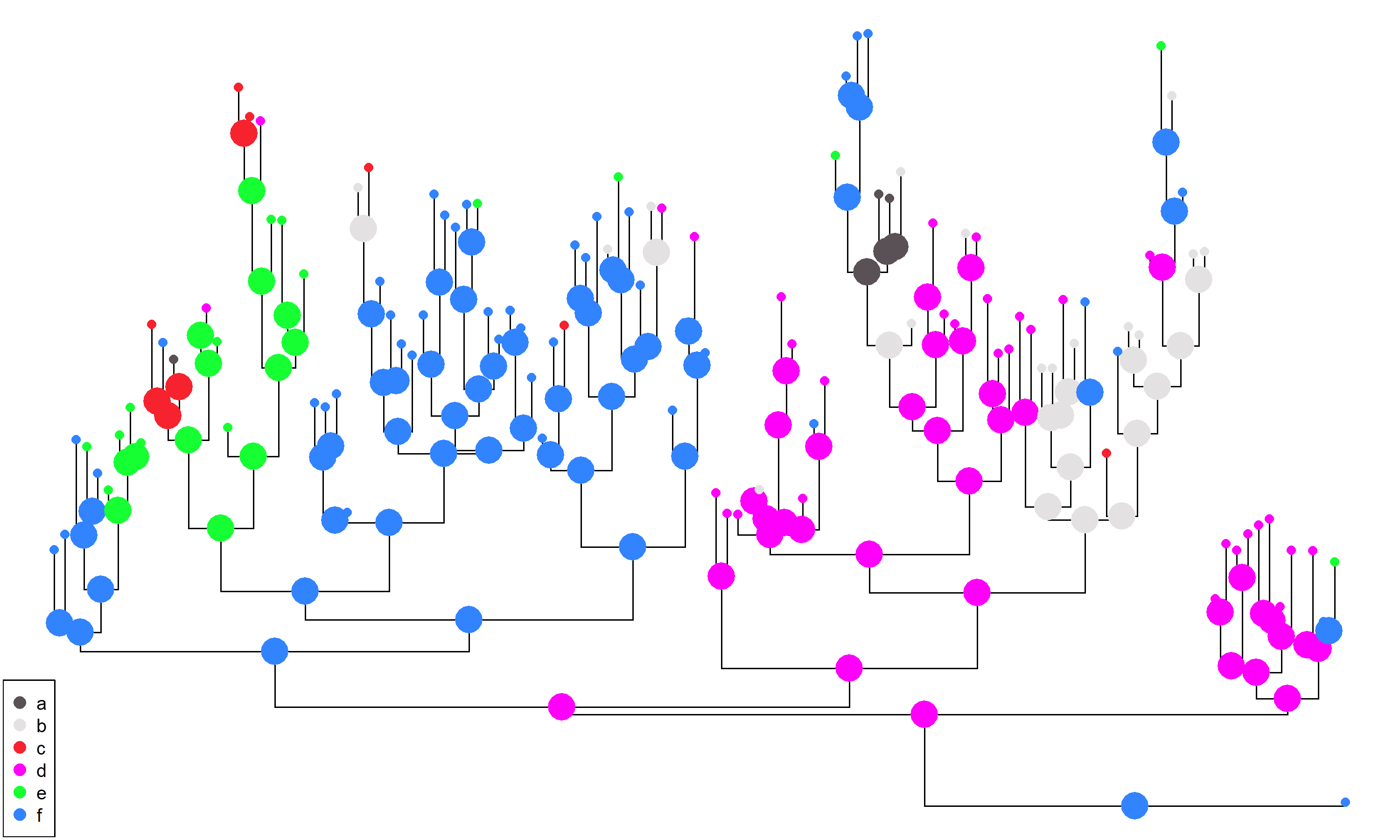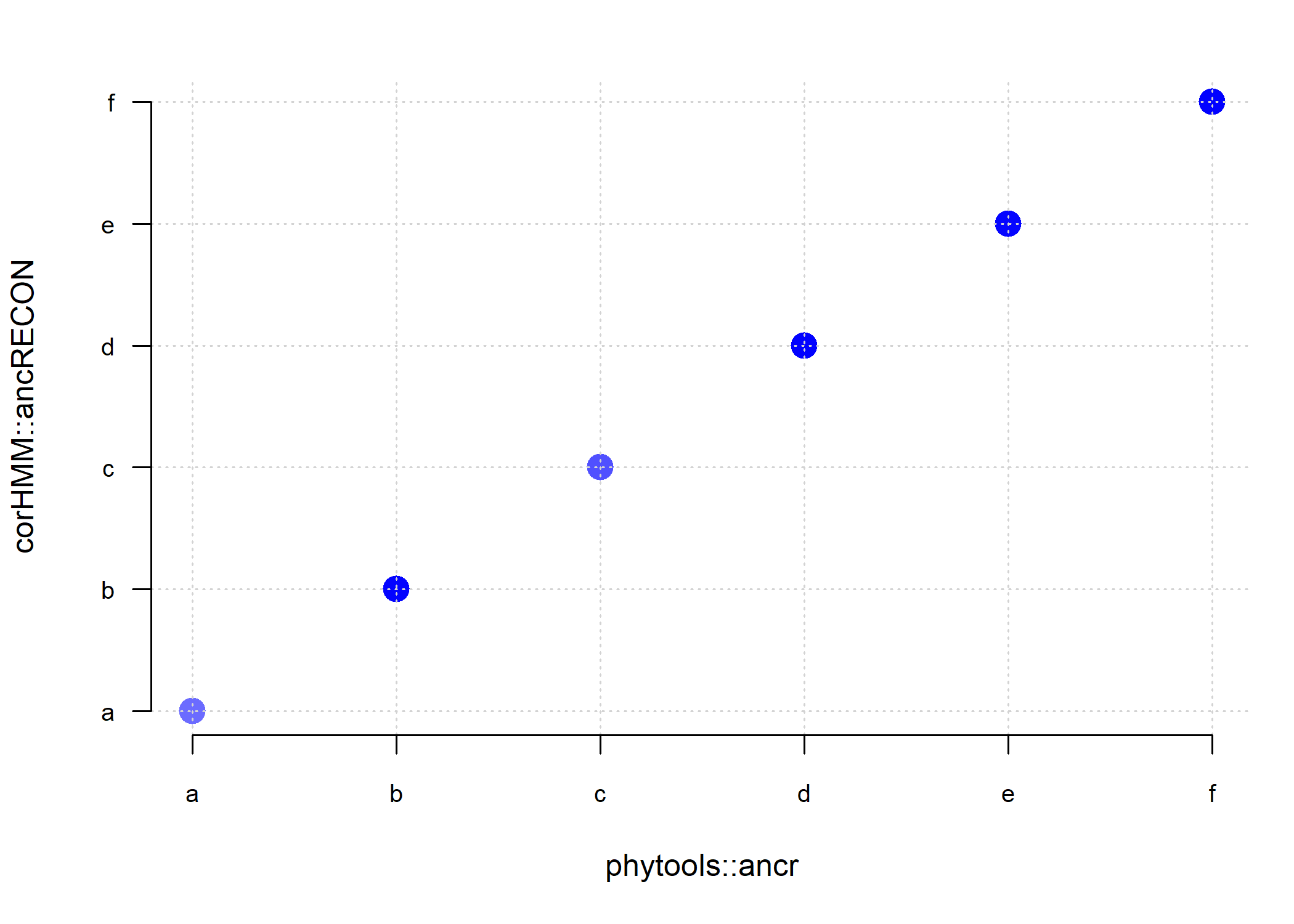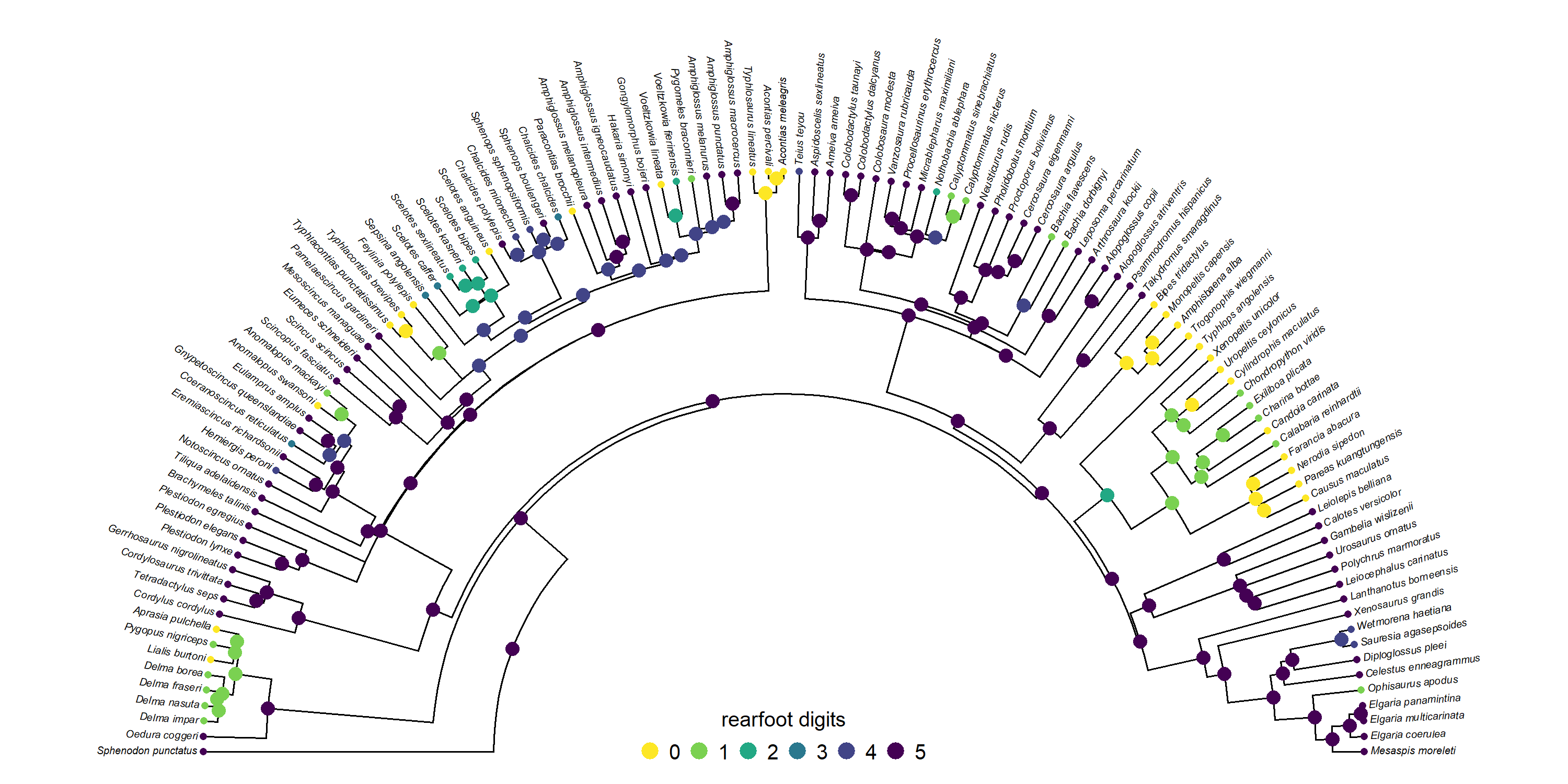## Sunday, May 14, 2023

### Joint ancestral state reconstruction of discrete characters in phytools

Little by little, I’ve been working on extending phytools’ functionality for ancestral character estimation.

Most recently, I added the generic method `ancr`, which can be used to compute marginal ancestral states from a fitted model object from `fitMk`, `fitHRM`, as well as `fitpolyMk` – and to do model averaging.

How I typically describe marginal ancestral state reconstruction is by imagining that we traverse the tree node by node. Each time we arrive to a node, we compute the probabilities (conditioned on our fitted model) that this node is in each of the different conditions of our state space, integrating over all possibilities for all other nodes of the tree.

Joint reconstruction, on the other hand, requires that we identify the set of states at all nodes of the phylogeny that, together, jointly maximize the probability of having obtained the set of states that we observed at the tips – once again, under our fitted model. Interesting, as pointed out by Yang (2006), the ancestral states with the highest marginal probabilities at all of the nodes of the tree (may, but) do not necessarily correspond to the set of states that jointly maximize the probability of our data: the joint reconstruction (Yang et al. 1995).

For comparative methods, the only R package that does joint ancestral state reconstruction is the excellent corHMM package of Jeremy Beaulieu et al.; however, Pupko et al. (2000) describe an efficient computational algorithm for joint reconstruction, so I thought I would just go ahead & try to implement it in phytools!

I’ve now added joint reconstruction as an option in the generic `ancr` method. For the time being, I’ll add the caveat that this is not yet thoroughly tested so I would encourage users to cross-check their findings using corHMM!

Let’s test it out using some simulated data as follows.

``````library(phytools)
``````
``````packageVersion("phytools")
``````
``````##  '1.9.1'
``````

Here, I’m going to create a random Q transition matrix for k = 6, and then simulated using this matrix.

``````k<-6
Q<-matrix(rexp(n=k*k,rate=10),k,k,dimnames=list(letters[1:k],letters[1:6]))
diag(Q)<-0
diag(Q)<--rowSums(Q)
x<-sim.Mk(tree<-rtree(n=120),Q)
``````

Now we can proceed to fit an all-rates-different (ARD) model with `phytools::fitMk`.

``````fitted_ard<-fitMk(tree,x,model="ARD",opt.method="optimParallel",
lik.func="pruning")
fitted_ard
``````
``````## Object of class "fitMk".
##
## Fitted (or set) value of Q:
##           a         b         c         d         e         f
## a -1.153436  0.627944  0.000315  0.000205  0.188650  0.336322
## b  0.000251 -1.024353  0.347650  0.539306  0.000026  0.137119
## c  0.616088  0.000340 -1.244101  0.071095  0.056933  0.499646
## d  0.000005  0.197928  0.000002 -0.334663  0.000001  0.136727
## e  0.000003  0.000039  0.211943  0.181347 -0.393334  0.000003
## f  0.000003  0.129348  0.000402  0.014668  0.197100 -0.341521
##
## Fitted (or set) value of pi:
##        a        b        c        d        e        f
## 0.166667 0.166667 0.166667 0.166667 0.166667 0.166667
## due to treating the root prior as (a) given.
##
## Log-likelihood: -134.227271
##
## Optimization method used was "optimParallel"
``````

Just passing this fitted model object to `ancr` and setting `type="joint"` should give us our result.

``````ard_ace<-ancr(fitted_ard,type="joint")
``````
``````## Note:
## 	 this option is new & I recommend cross-checking
``````
``````ard_ace
``````
``````## Joint ancestral state estimates:
##     state
## 121     f
## 122     d
## 123     d
## 124     f
## 125     f
## 126     f
## ...
##
## Log-likelihood = -140.35541
``````

We see that the finding prints as a set of reconstructions at internal nodes – this is the set that makes our data at the tips most probable, under our fitted transition model.

Let’s plot them on the tree as follows.

``````plot(ard_ace,args.plotTree=list(direction="upwards",ftype="off"))
``````This result can be pretty easily compared to what we might have obtained using corHMM. To avoid re-fitting my model, I’m going to use the handy function `ancRECON`. This will allow be to supply the parameters of my fitted model generated using `fitMk`.

``````library(corHMM)
``````
``````MODEL<-fitted_ard\$index.matrix
DATA<-data.frame(Genus_sp=names(x),X=x)
ard_corhmm<-ancRECON(tree,DATA,fitted_ard\$rates,"joint",
rate.cat=1,rate.mat=MODEL,root.p=fitted_ard\$pi)
ard_corhmm
``````
``````## \$lik.tip.states
##    6 6 6 5 6 5 5 5 5 3 6 1 3 5 4 5 5 3 3 4 5 5 5 5 6 6 6 6 2 3 6 6 6 6 6 6 6 6 6 5 6 6 6 6 6 6 6 3
##   6 6 6 2 5 6 6 2 4 6 6 4 6 4 4 4 4 2 4 4 4 4 6 4 5 6 6 6 1 1 2 2 4 4 4 4 2 4 4 4 4 4 4 2 2 4 2 6
##   6 3 6 2 2 4 5 2 6 2 2 4 4 4 4 4 4 4 4 4 4 6 5 6
##
## \$lik.anc.states
##    6 4 4 6 6 6 6 6 6 5 5 5 6 6 5 5 3 3 3 5 5 5 5 5 5 3 5 5 6 6 6 6 6 6 6 6 2 6 6 6 6 6 6 6 6 6 6 6
##   6 6 6 6 6 6 6 6 6 6 6 2 6 6 6 4 4 4 4 4 4 4 4 4 4 4 4 4 4 4 2 1 6 6 6 1 1 4 4 4 4 4 4 2 2 4 2 2
##   2 2 6 2 2 2 2 2 4 6 6 2 4 4 4 4 4 4 4 4 4 4 6
``````

`ancRECON` has recoded our data numerically, but this will be helpful in comparing these results to those that we obtained via `ancr`, so there’s no need to translate them back.

``````par(mar=c(5.1,4.1,2.1,1.1))
plot(as.numeric(ard_ace\$ace),ard_corhmm\$lik.anc.states,bty="n",
pch=16,col=make.transparent("blue",0.25),cex=2,axes=FALSE,
xlab="phytools::ancr",ylab="corHMM::ancRECON")
grid()
axis(1,at=1:k,labels=letters[1:k],las=1,cex.axis=0.8)
axis(2,at=1:k,labels=letters[1:k],las=1,cex.axis=0.8)
``````Awesome! It seems we haven’t done half bad.

Now let’s try a “real” example: squamate digit number using our trusty old toe number data adapted from Brandley et al. (2008).

``````library(geiger)
row.names=1)
toe_number<-as.factor(setNames(squamate.data[],rownames(squamate.data)))
chk<-name.check(squamate.tree,squamate.data)
squamate.tree<-drop.tip(squamate.tree,chk\$tree_not_data)
toe_number<-toe_number[squamate.tree\$tip.label]
``````

Fit our extended Mk model

``````model<-matrix(c(
0,6,0,0,0,0,
1,0,7,0,0,0,
0,2,0,8,0,0,
0,0,3,0,9,0,
0,0,0,4,0,10,
0,0,0,0,5,0),6,6,byrow=TRUE)
toe_ordered<-fitMk(squamate.tree,toe_number,model=model,pi="fitzjohn",
opt.method="optimParallel",lik.func="pruning")
``````

Compute our joint reconstruction.

``````toe_anc<-ancr(toe_ordered,type="joint")
``````
``````## Note:
## 	 this option is new & I recommend cross-checking
``````
``````toe_anc
``````
``````## Joint ancestral state estimates:
##     state
## 120     5
## 121     5
## 122     5
## 123     5
## 124     5
## 125     5
## ...
##
## Log-likelihood = -132.16474
``````

Finally, plot our results.

``````cols<-setNames(viridisLite::viridis(n=6,direction=-1),0:5)
plot(toe_anc,args.plotTree=list(type="arc",arc_height=1,fsize=0.4),
args.nodelabels=list(piecol=cols),legend=FALSE)
legend(x=0,y=40,0:5,pch=16,cex=0.8,pt.cex=1.5,col=cols,
horiz=TRUE,xjust=0.5,bty="n",title="rearfoot digits")
``````Makes sense.